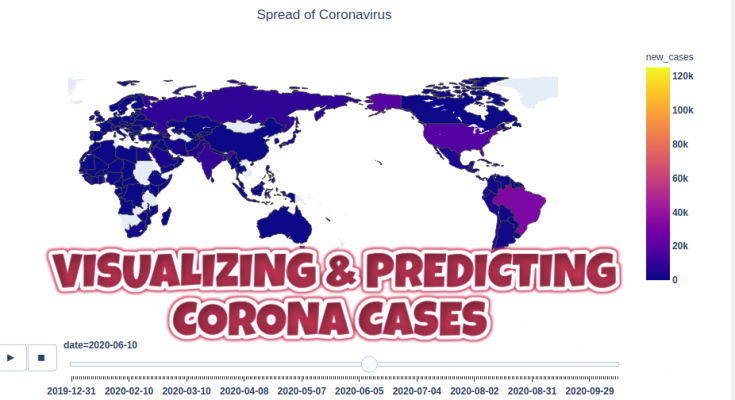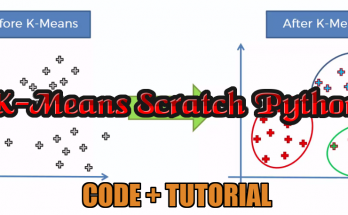# VISUALIZING & PREDICTING CORONA CASES – LATEST AI PROJECTFrom World Health Organization – On 31 December 2019, WHO was alerted to several cases of pneumonia in Wuhan City, Hubei Province of China. Since then Corona Virus is spreading across the globe. In this project ” VISUALIZING & PREDICTING CORONA CASES”, we will be using a dataset from Our World in Data.

Here we will be visualizing the current trend of cases and trends in Asia, especially in Nepal(You can choose your own country). So, let’s begin the project by importing the dataset & other libraries.

Let’s see how Corona Spread VS Time :

#### VISUALIZING CASES IN ASIA REGION

Here, we are visualizing the corona datasets only from Asia. So, we will be selecting datasets related to the Asia continent only. We will be grouping the datasets by country name i.e location and date. Now, we will visualize the cureent corona cases in the Asia region as of October 13.

As the corona cases in India is too large comparing with other countries, we couldn’t visualize the corona cases in all countries of Asia. So, we will be removing India from the list and will visualize again.

#### VISUALIZING & PREDICTING CORONA CASES IN NEPAL

In the above dataset, we have lots of columns(feature labels). We aren’t using all of them in our project so we will select only the required columns from our dataset. So let’s make it clear at first: We will be using new cases, total cases, new deaths, and total cases from Nepal.

#### PREDICTING FUTURE CORONA CASES IN NEPAL

Since the number of cases for the first few days is very small, there is a large fluctuation in the early part of the graph, after which it stabilizes to a nearly straight line. Hence, we choose to ignore the low valued-data in order to develop a model with a better fit. We will also be adding Serial Number i.e SN on the dataset in today’s project of

##### PREPARING DATASET

Here, to predict the future Corona Cases in Nepal, we will be using Linear Regression. We will be calculating the accuracy with various degree best fit line curve equation.

Regression is the method which measures the average relationship between two or more continuous variables in term of the response variable and feature variables. In other words, regression analysis is to know the nature of the relationship between two or more variables to use it for predicting the most likely value of dependent variables for a given value of independent variables. Linear regression is a mostly used regression algorithm.

```----------------------polynomial model training--------------------
accuracy(R2) with degree_1 is -->  86.37%
accuracy(R2) with degree_2 is -->  99.155%
accuracy(R2) with degree_3 is -->  99.778%
accuracy(R2) with degree_4 is -->  99.779%
accuracy(R2) with degree_5 is -->  99.935%
-------------------------------------------------------------------```

Now, we will be choosing polynomial degree as 4 and we will plot actual vs predicted graph.

##### PREDICTING CORONA CASES BY DAYS
```>>> #prediction after 7 days
>>> days = 7
>>> print("Corona Cases after {} day - ".format(days), end='')
>>> print(round(int( model.predict(polyfet.transform (np.array(x1[-1:] +days).reshape(-1,1)))),2))

Output:- Corona Cases after 7 day - 125281```

##### AI FROM SCRATCH FREE COURSE
View All### K-Means Clustering From Scratch Python – Free Machine Learning Course

KNN ##### In this article, we will cover k-means clustering from scratch. In general, Clustering is defined as the grouping of data points such that the data points in a …

### RANDOM FOREST FROM SCRATCH PYTHON